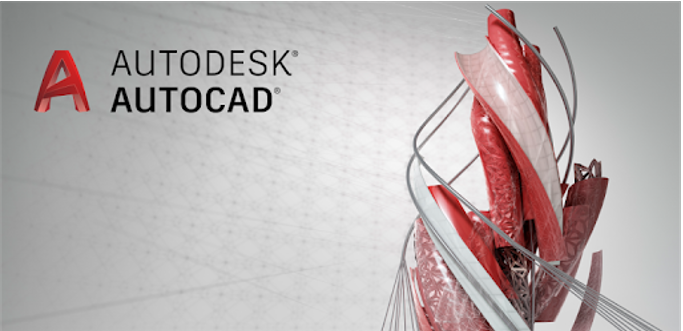Autocad shortcuts from Autodesk

### DRAWING

• L     = line / Creates straight line segments .
• C    =Circle /  Creates a circle
• PL  =Polyline / Creates a 2D polyline
• POPOINT / Creates a point object
• ML = Multi lِine
• X    = CONSTRUCTION LIِNE
• REC = Rectangle / Creates a rectangular polyline
• BO   = Boundary / Creates a region or a polyline from an enclosed area
•  = Hatch / Fills an enclosed area or selected objects with a hatch pattern, solid fill, or gradient fill
• A = ARC / Creates an arc
• AAAREA / Calculates the area and perimeter of objects or of defined areas

### MODIFY

• CO    = Copy  / Copies objects a specified distance in a specified direction
• O       = OFFEST / Creates concentric circles, parallel lines, and parallel curves
• MI     = Mirror / Creates a mirrored copy of selected objects
• B      = BLOCK / Creates a block definition from selected objects
• SC   = Scale / Enlarges or reduces selected objects, keeping the proportions of the object the same after scaling
• REG  = REGION / Converts an object that encloses an area into a region object
• BO   = Boundary / Creates a region or a polyline from an enclosed area
• J       = JOIN    / Joins similar objects to form a single, unbroken object
• M     = MOVE   / Moves objects a specified distance in a specified direction
• EX   = EXTEND / Extends objects to meet the edges of other objects
• TR   = TRIM / Trims objects to meet the edges of other objects
• X  + ENTER     = EXPLOUDE / Breaks a compound object into its component objects.
• DI + ENTER     = DISTANCE / Measures the distance and angle between two points
• DIM + ENTER  = DIMENSION / Displays property data for selected objects
• LI      = LIST / Displays property data for selected objects
• RO    = ROTATE / Rotates objects around a base point
• AR    = ARRAY / Creates multiple copies of objects in a pattern
• F       = FILLET / Rounds and fillets the edges of objects
• BR    = BREAK / Breaks the selected object between two points